# Pandas Map Dictionary values with Dataframe Columns

Pandas has a cool feature called Map which let you create a new column by mapping the dataframe column values with the Dictionary Key. Let’s understand this by an example:

Create a Dataframe:

Let’s start by creating a dataframe of top 5 countries with their population

``````import pandas as pd
df= pd.DataFrame({'Country':['China','India','USA','Indonesia','Brazil'],'Population':[1403500365,1324171354,                                                                                     322179605,261115456,                                                                                     207652865]})
``````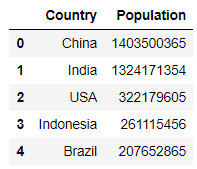Create a Dictionary

This dictionary contains the countries and their corresponding National capitals, Where country is the Key and Capital is the value

``````country_capital=
{
'Germany':'Berlin',
'Brazil':'Brasília',
'Budapest':'Hungary',
'China':'Beijing',
'India':'New Delhi',
'Norway':'Oslo',
'France':'Paris',
'Indonesia': 'Jakarta',
'USA':'Washington'
}
``````

Now we have a dataframe of top 5 countries and their population and a dictionary which holds the country as Key and their National Capitals as value pair. Let’s create a new column called capital in the dataframe matching the Key value pair from the country column

Create Column Capital matching Dictionary value

``````df['Capital'] = df['Country'].map(country_capital)
``````

Voila!! So we have created a new column called Capital which has the National capital of those five countries using the matching dictionary value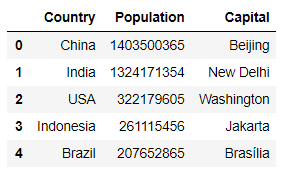Map Accepts a Function Also

Let’s multiply the Population of this dataframe by 100 and store this value in a new column called as inc_Population

``````df['inc_Population']=df.Population.map(lambda x: x*100)
``````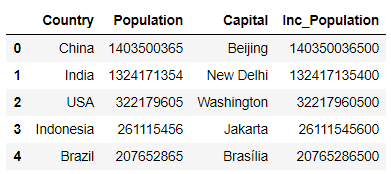# Pandas Replace from Dictionary Values

We will now see how we can replace the value of a column with the dictionary values

Create a Dataframe

Let’s create a dataframe of five Names and their Birth Month

``````df= pd.DataFrame({'Name':['Allan','John','Peter','Brenda','Sandra'],'birth_Month':[5,3,8,12,2]})
``````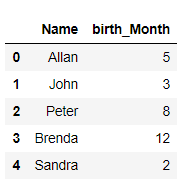Create a Dictionary of Months

Let’s create a dictionary containing Month value as Key and it’s corresponding Name as Value

``````Month_Values=
{
1:'January',
2:'February',
3:'March',
4:'April',
5:'May',
6:'June',
7:'July',
8:'August',
9:'September',
10:'October',
11:'November',
12:'December'
}
``````

Replace

Let’s replace the birth_Month in the above dataframe with their corresponding Names

``````df['birth_Month'] = df.birth_Month.replace(country_capital)
``````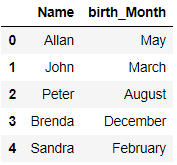# Pandas Update column with Dictionary values matching dataframe Index as Keys

We will use update where we have to match the dataframe index with the dictionary Keys

Lets use the above dataframe and update the birth_Month column with the dictionary values where key is meant to be dataframe index, So for the second index 1 it will be updated as January and for the third index i.e. 2 it will be updated as February and so on

``````df.birth_Month.update(pd.Series(country_capital))
``````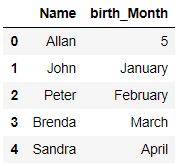There is no matching value for index 0 in the dictionary that’s why the birth_Month is not updated for that row and all other rows the value is updated from the dictionary matching the dataframe indexes

Tags:

Categories:

Updated: